#### 前言

• 对两种正则化方法的理解总结

#### 过拟合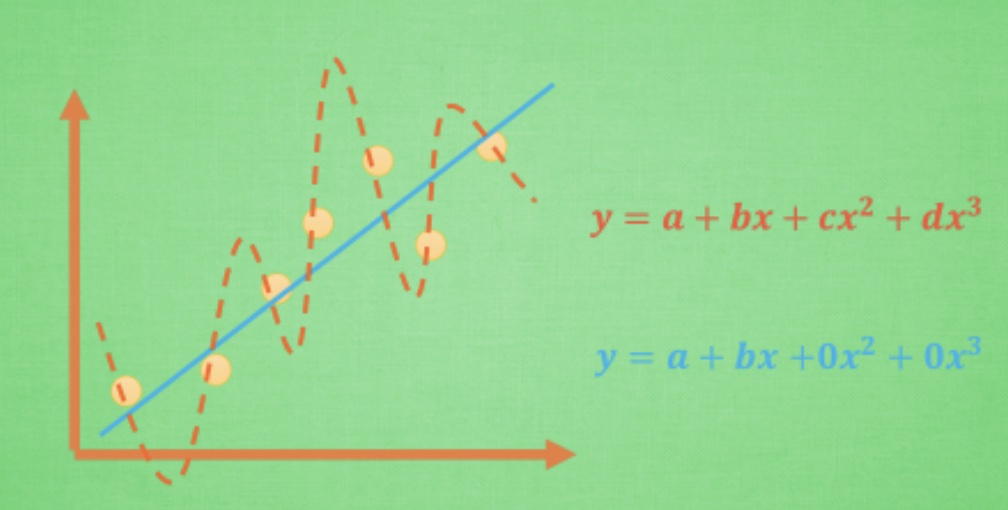#### L1和L2正则化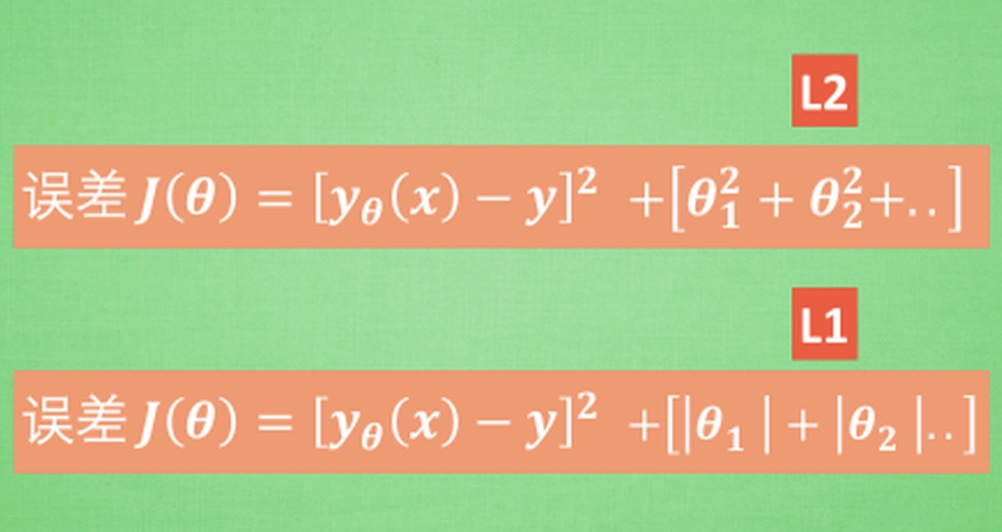L1范数：$|x|{1}=\left|x{1}\right|+\left|x{2}\right|+\left|x{3}\right|+\cdots+\left|x_{n}\right|$

L1 公式：$J = loss + \sum\lambda ||w||$

L2范数：$|x|{2}=\left(\left|x{1}\right|^{2}+\left|x{2}\right|^{2}+\left|x{3}\right|^{2}+\cdots+\left|x_{n}\right|^{2}\right)^{1 / 2}$

L2 公式：$J = loss + \sum\lambda ||w||_2^2$ 符号：$|w|$表示向量的模，外面再加一层||代表绝对值，没有特别含义；$|w|_2$下面的2代表开根号

#### 主要思想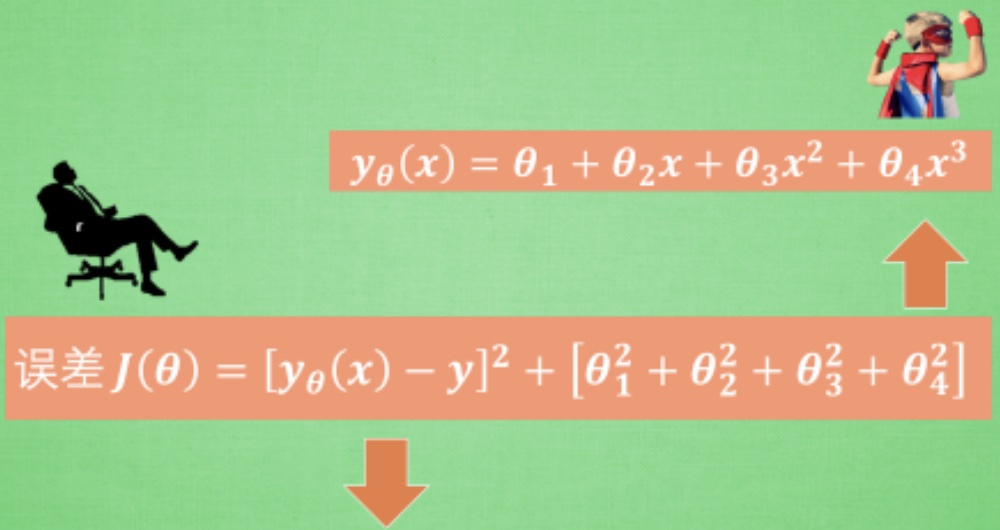$\theta_4$ 说, 瞧我本事多大, 就让我来改变模型, 来拟合所有的数据吧, 可是它这种态度招到了误差方程的强烈反击, 误差方程就说: no no no no, 我们是一个团队, 虽然你厉害, 但也不能仅仅靠你一个人, 万一你错了, 我们整个团队的效率就突然降低了, 我得 hold 住那些在 team 里独出风头的人. 这就是整套正规化算法的核心思想

#### 结构风险最小化角度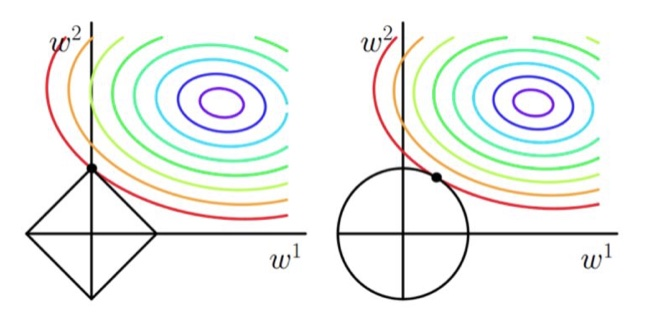• 如果不加L1和L2正则化，对于线性回归这种目标函数凸函数的话，最终的优化的结果就是最里边的紫色的小圈圈等高线上的点

• 当加入L1正则化的时候，我们先画出 $|w_1| + |w_2| = F$ 的图像，也就是一个菱形，代表这些曲线上的点算出来的 1范数 $|w_1| + |w_2|$ 都为F。那我们现在的目标是不仅是原曲线算得值要小（越来越接近中心的紫色圈圈），还要使得这个菱形越小越好（F越小越好)。那么还和原来一样的话，过中心紫色圈圈的那个菱形明显很大，因此我们要取到一个恰好的值。那么如何求值呢？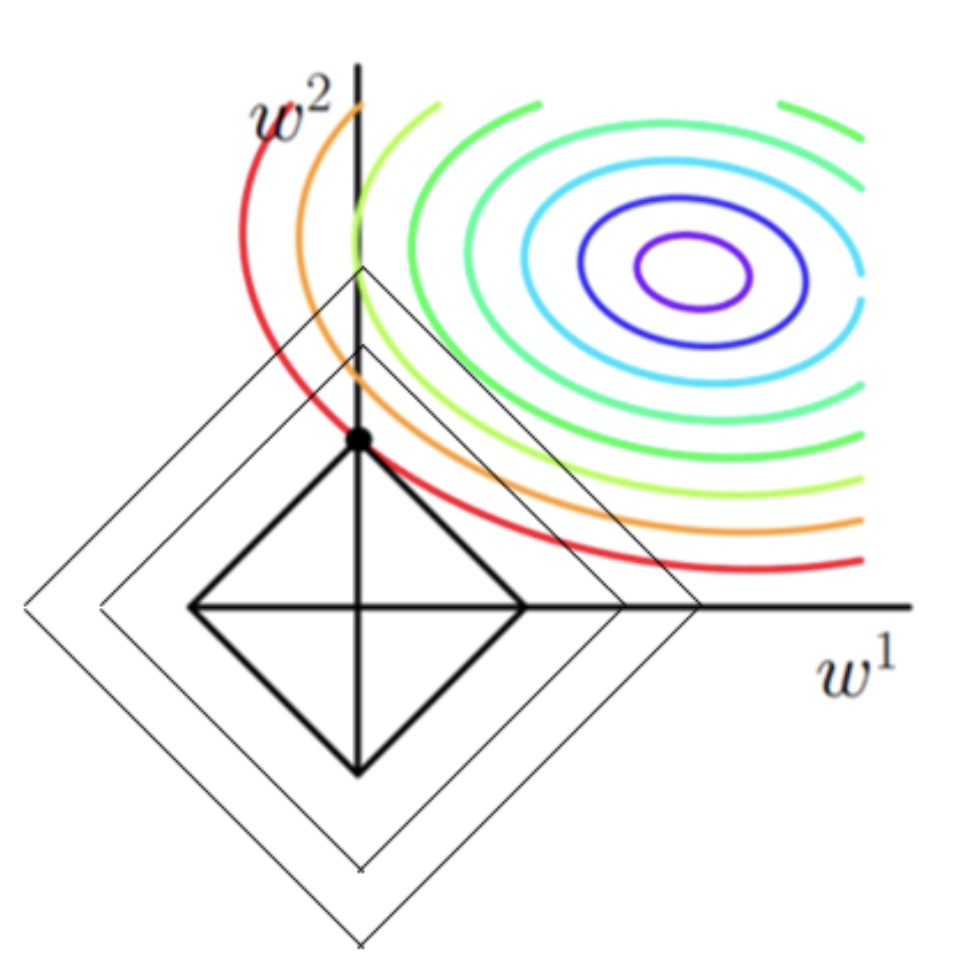-------------The End-------------